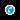# Finite Element Method

Welcome to my first post here. Let’s begin the discussion about the Importance of Finite element Analysis. Now the reason I chose to discuss analysis prior to 3D modeling is that Analysis is the end of the whole design process.  If your product fails in Analysis, then you have to restructure the product along with its material, depends on the particular condition. Hence consideration of analysis while modeling is of utmost importance. Analyzing the product assures the authenticity of predefined assumptions and further clears the path to make changes in order to optimize the product. At AutoCAD Classes in Pune, you will get to know more about FEM and its importance in the analysis. In analytical methods, Finite element methods are widely used for reasons we will discuss in detail below.

Ever since the evolution of science, we are concentrating on the study of a matter, digging it to the cellular level. Over the years now we have learned that such tiniest particles behave in a particular manner under the particular situation, making a property of a material.  Dividing a matter into tiny parts, we get to observe its nature across different conditions. Finite element analysis works on a similar principle, dividing a product into a number of elements to examine its properties in different situations. This method is called a method of discretization.  In engineering analysis, there are complex structures working in extreme natural/artificial environments. Such complex structures are hard to examine as a whole, hence scientists started the method of discretization. After discretization, the structure needs to be examined analytically. For analytical methods, FEA uses differential equations. Before proceeding further, let’s talk about differential equations and their significance in Finite element analysis for a while.

In mechanical engineering analysis, differential equations are used to address the governing equations through different variables. The differential equation relates multiple functions with their derivatives in equation form. Derivatives are differentiated with respect to variables, in other words, it is the rate of change of an entity with respect to any (in general one of the fundamental physical property) physical property.

Let’s consider a very basic example for better clarification:

We know the spring force equation is F=-k.x

Where k=spring constant and x is the displacement of a spring.

And as per newton’s second law force F= m.a, Where m= mass and a= acceleration (Gravity for free falls)

Therefore,

m.a = -k.x

Acceleration is a second-order derivative of distance.

Therefore we can rewrite above equation as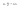This is called as a differential equation. Here variable and it’s derivative both present in an equation.

The most common differential equations used in FEM are Partial differential equations (PDE).

Partial differential equations contain multivariable functions which are derived in turns to obtain the desired function.  In FEM, PDE is used to present the dependency of an entity over other entities described in equation form. To understand it better, consider the example of heat transfer. Now, Heat is a function of time as well as space. Time and space both simultaneously represents the heat transfer across the object. So here Time and space are variables and change in heat is a function domain.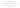Here, derivatives of temperature with respect to x are second-order derivative. That means it is the rate of change of temperature in space. In short, it is a deflection in slope. Such many more equations from different applications are represented in partial differential equations.

For complex geometries, it is impossible to solve the equations analytically.  Hence these equations are approximated with numerical model equation after discretization. FEM tries to solve such equations.

After discretization, boundary or initial conditions need to be provided. While solving PDEs, the necessary inputs are evaluated based on the type of equation.  In mathematical terms, boundary conditions are expressed as a boundary value problem that contains a set of constraints. Finding the solution to these boundary value problems satisfy the boundary condition.  PDEs are categorized, parabolic, elliptic, and hyperbolic. Elliptic PDE has two approaches, Finite difference method, and the variation energy approach.

Finite difference method:

To get the approximate value of an equation we use numerical methods like newton-Raphson, Runge-Kutta, etc. Finite difference methods are the type of partial differential numerical methods that provide approximate solutions or formulas to find the solutions.

The beauty of FDM is that it can be applied to find out approximate values in 2D space by providing inputs as boundary conditions. By changing these inputs and after running multiple iterations, we get the range of solutions across the desired region. In terms of software, on a window to consider practical applications, there is a grid used as a reference by FDM. FDM represents field properties on the grid with the help of different formulas. By using difference formula we get the value of an entity at the particular instance and value of the same entity at immediately next instance. Then we calculate the rate of difference between the two. Results we get are as algebraic equations, usually in the form of the matrix. The solution of this matrix is the approximate solution to the PDE.  The advantage of FDM is that it can be applied to any PDEs.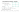From the above example in image 1, we can see that there is a total of 9 nodes of a grid, let’s say 9 instances. Node.js represents reference instances and Nodei+1 and Nodej+1 are immediate next instances over the space. Similarly, Ni-1 and Nj-1 are previous once. Using the poisons equation we can use the second-order derivative to calculate approximate values. Formula to discretize the terms in x and y direction respectively is shown. g represents the constant and depends on the application it is used. Let’s consider the heat transfer along the rod. Then temperature profile long the rod will be calculated using the above equations and g can be taken as the heat source.

Due to its usefulness, FDM is popular in Heat transfer, Fluid dynamics, acoustics, etc.

Finite Volume methods:

The finite volume method is a numerical technique to get the approximate solution to PDEs. FVM expresses the conservation of one or more quantities. FVM discretizes the part or system by dividing it into specific control volumes in the form of mesh. The result can become more accurate by refining the mesh. Control volumes are separated by mesh grid lines. The integration of the governing equations over the control volume results in balance equations. This is equivalent to applying basic conservation law to each control volume. Discretization involves the substitution of a variety of finite difference type approximations for the terms in the integrated equations resulting in inflow processes such as convection, diffusion, and sources. This converts integral equations in the algebraic once. By iterations, the solution to this algebraic equation is found out.FVM needs only to evaluate flux at cell boundaries. This allows an evaluation of even non- linear boundaries and makes it eligible for robust handling of conservation laws. In FVM, the function that approximates the solution cannot be easily made of higher-order, making It a disadvantage of FVM. FVM is popular for solving fluid flow problems successfully. At SevenMentor’s Auto CAD Classes in Pune, you get to know a Fluent solver that uses FVM.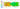As discussed, in FEM the solution domain is discretized into smaller regions, aka finite elements. For approximation functions, interpolation functions are assumed. These functions represent the variables over finite elements. Same as FDM and FVM, a set of governing differential functions are integrated with some weighting functions over all elements. It produces a set of algebraic equations in matrix form. To compare between FDM, FVM, and FEM, each method has its own way to represent a systematic numerical method for solving Partial differential equations. But they differ in way of implementing efficiently and easily. FVM provides better approximation over non-linear functions than others. FDM is the easiest method amongst three to implement. Finite element Analysis is popular for structural and electromagnetic analysis. In both these fields of physics approximated accurately as FEM allows increasing the order of elements. This corresponds to the use of polynomials of higher-order, the order of more than 2. It results in an inaccurate analysis of stresses. One more advantage of FEM is that when there are critical areas to examine like corners where stress concentration can be more, FEM allows refining the mesh locally to that particular area, increasing the number of elements. Finer the mesh, more accurate the result.

FEM allows for mixed formulations. It means that FEM can combine different kinds of functions.

For example, let’s assume the case of electromagnetic heating. The mathematics and physics laws required one for electromagnetics and another for heat transfer. Both functions need to be coupled in order to converge the solution. Mixed formulations are easily compatible with FEM.

Using all the above 3 methods after solving the algebraic equations, we get the results over the specified control regions. This result is then displayed in tabular format after solving the problem analytically. In tabular form, it is easy to access different data from different regions.

## Call the Trainer and Book your free demo class for now!!!